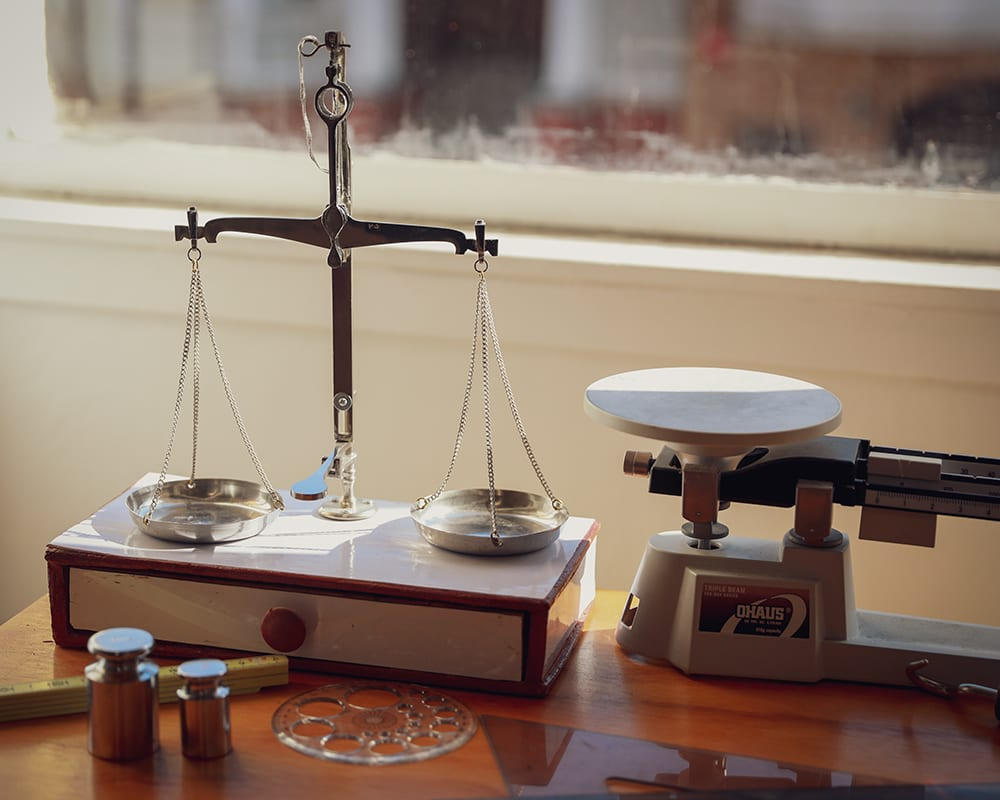#### Middle School Mathematics DepartmentMath comes from daily life. We encourage students to learn by investigation and become creative problem solvers in real-to-life mathematical scenarios. Classes in lower grade-levels incorporate foundational skills in algebra and strategic reasoning. Upper-grade levels explore advanced areas of math and their real-world applications.

The comprehensive program includes a range courses from Algebra I and Geometry to Calculus, Statistics, and Discrete Math. Students learn to develop independent thinking, connect visuals to algebraic representations, and become more confident with their ability to reason logically.

#### Course Description

• Math 6
• Pre-Algebra
• Algebra I

Prerequisites: None

In this sixth grade class, students will build on the mathematical skills they learned in elementary school and prepare for pre-algebra the following year. Some topics include: reviewing division by whole numbers and by fractions, using chains of equivalent terms to write calculations in different ways, understanding ratios and rates, describing patterns with graphs, understanding negative numbers, using percentages, and analyzing mathematical data.

Prerequisites: None

In this seventh grade class, students will prepare for algebra the following year. Some topics include: understanding advanced decimals and percentages, studying negative and positive fractions, describing mathematical and real-life situations using variables, understanding tables, graphs and equations, performing constructions and calculations with triangles, other polygons, and circles, determining scales and distances related to maps, using data and probability calculations to make predictions, and calculating the surface area and volume of various solids.

Prerequisites: None

This course is offered to students who have completed pre-algebra, and is typically taken in 8th grade or 9th grade. Students in this course will learn to describe patterns using words, variables, and chains of equivalent terms, study the characteristics of irrational numbers, practice calculations with roots, differentiate between linear and exponential growth, as well as arithmetic and geometric sequences, and use the power properties to simplify expressions, write and solve equations and inequalities, and use quadratic functions.

#### Mathematics Department Faculty### Ms. Cornelia Ritter

Math Teacher
Ms. Ritter obtained a Masters degree in Physics from the University of Bern, with a minor in mathematics. In 1997, she received her teaching credentials for secondary schools in mathematics and physics. She has more than 20 years of teaching experience.### Mr. Ray Chen

Science Teacher
Mr. Ray Chen received his Master of Science degree from Purdue University. He brings decades of work experience from global enterprises like Apple and Oracle, as well as years of experience from teaching previously at High School of the Arts and at other private schools.